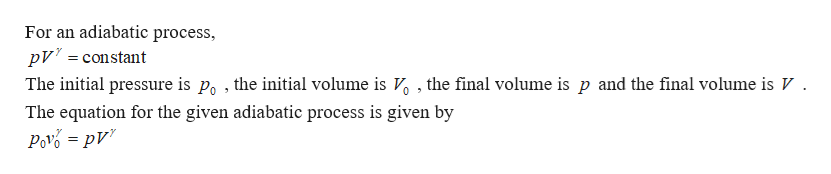# In this problem you are to consider an adiabaticexpansion of an ideal diatomic gas, which means that the gas expands with no addition or subtraction of heat.Assume that the gas is initially at pressure p0, volume V0, and temperature T0. In addition, assume that the temperature of the gas is such that you can neglect vibrational degrees of freedom. Thus, the ratio of heat capacities is γ=Cp/CV=7/5.Note that, unless explicitly stated, the variable γshould not appear in your answers--if needed use the fact that γ=7/5 for an ideal diatomic gas. Find an analytic expression for p(V), the pressure as a function of volume, during the adiabatic expansion.Express the pressure in terms of V and any or all of the given initial values p0, T0, and V0. p(V) = __________

Question
66 views

In this problem you are to consider an adiabaticexpansion of an ideal diatomic gas, which means that the gas expands with no addition or subtraction of heat.

Assume that the gas is initially at pressure p0, volume V0, and temperature T0. In addition, assume that the temperature of the gas is such that you can neglect vibrational degrees of freedom. Thus, the ratio of heat capacities is γ=Cp/CV=7/5.

Note that, unless explicitly stated, the variable γshould not appear in your answers--if needed use the fact that γ=7/5 for an ideal diatomic gas.

Find an analytic expression for p(V), the pressure as a function of volume, during the adiabatic expansion.

Express the pressure in terms of V and any or all of the given initial values p0, T0, and V0.

p(V) = __________
check_circle

star
star
star
star
star
1 Rating
Step 1help_outlineImage TranscriptioncloseFor an adiabatic process, pV constant The initial pressure is po , the initial volume is V , the final volume is p and the final volume is V The equation for the given adiabatic process is given by Pov=pv fullscreen

### Want to see the full answer?

See Solution

#### Want to see this answer and more?

Solutions are written by subject experts who are available 24/7. Questions are typically answered within 1 hour.*

See Solution
*Response times may vary by subject and question.
Tagged in

### Physics